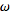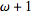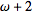# What is before the first transfinite ordinal, omega?

• I
• zrek
In summary, the conversation discusses the concept of transfinite ordinals and the notation used to represent them. It is mentioned that Omega is the first transfinite ordinal and is followed by other transfinite ordinals. However, when asked what comes before Omega, there is no clear answer as the notation does not work in reverse. Therefore, it is concluded that there is nothing before Omega in the set of transfinite ordinals.

#### zrek

Omega is the first transinite ordinal in the set of 0, 1, 2, ...,,,, ...
This set is well ordered, sois afterthereforeis before.

What is before?

Options:
1.-1
This is confusing, since ifis the first transfinite ordinal, then-1 should be the last finite ordinal, which simply does not exist.
2. Undefined
3. Nothing (just like in the set of natural numbers (N1), there is nothing before one)

Please don't use images for greek characters ... this site supports LaTeX markup.
##0,1,2,\cdots, \omega , \omega +1, \omega + 2, \cdots , \omega+\omega, \omega + \omega + 1, \cdots## ... no images used see?

What is before ##\omega##
Please define your terms - in doing so, you need to reference the definition of "transfinite number".
What do you mean by "before"?

i.e. the set of ordinals less than the 1st transfinite number could be the set of ordinals excluding all transfinite ordinals... this would be the set of whole numbers.
Are you asking for the highest whole number?

But I suspect you may just be getting mislead by the notation... consider: ##1+\omega = \omega## but ##\omega + 1 > \omega##
Thus: ##^-1+\omega = \omega## but ##\omega - 1## doesn't mean anything.

zrek said:
Omega is the first transinite ordinal in the set of 0, 1, 2, ..., [PLAIN]http://mathworld.wolfram.com/images/equations/OrdinalNumber/Inline5.gif, [Broken] [PLAIN]http://mathworld.wolfram.com/images/equations/OrdinalNumber/Inline6.gif, [Broken] [PLAIN]http://mathworld.wolfram.com/images/equations/OrdinalNumber/Inline7.gif, [Broken] ...
This set is well ordered, sois afterthereforeis before [PLAIN]http://mathworld.wolfram.com/images/equations/OrdinalNumber/Inline7.gif. [Broken]

What is before?

Options:
1. [PLAIN]http://mathworld.wolfram.com/images/equations/OrdinalNumber/Inline5.gif-1 [Broken]
This is confusing, since ifis the first transfinite ordinal, then [PLAIN]http://mathworld.wolfram.com/images/equations/OrdinalNumber/Inline5.gif-1 [Broken] should be the last finite ordinal, which simply does not exist.
2. Undefined
3. Nothing (just like in the set of natural numbers (N1), there is nothing before one)

Good question. The answer is that there is no transfinite number right before ##\omega##. We say that ##\omega## is a limit ordinal. It can only be reached through a limit.

However, you can extend the system of transfinite numbers to form the so called surreal numbers. These form a huge number field. In this number field, something like ##\omega-1## does exist.

Last edited by a moderator:
•Simon Bridge
Simon Bridge said:
i.e. the set of ordinals less than the 1st transfinite number could be the set of ordinals excluding all transfinite ordinals... this would be the set of whole numbers.
Are you asking for the highest whole number?
Omega used to (axiomatically) symbolize the first transfinite ordinal in the field of transfinite set theory. Yes, I know that "before" it there lies the complete set of the natural numbers, but if we accept the notation and existence of ##\omega +1## and the whole set is well ordered, including the transit area between the finite and transfinite parts, then we should have some definition for ##\omega -1## too.

micromass said:
Good question. The answer is that there is no transfinite number right before ##\omega##. We say that ##\omega## is a limit ordinal. It can only be reached through a limit.
(I think that your answer means that the option 2, the "undefined" is the correct answer, right?)

I wonder how the transfinite set theory meant to handle this exactly. (I don't want to step forward to surreal numbers)
If there is no transfinite number right before ##\omega##, then what is more correct to say:
1. there is something before it, but it can not be defined, or
2. there is nothing right before it (so the well ordered continueness does not work backwards)
3. other?

zrek said:
(I think that your answer means that the option 2, the "undefined" is the correct answer, right?)

I wonder how the transfinite set theory meant to handle this exactly. (I don't want to step forward to surreal numbers)
If there is no transfinite number right before ##\omega##, then what is more correct to say:
1. there is something before it, but it can not be defined, or
2. there is nothing right before it (so the well ordered continueness does not work backwards)
3. other?

2 would be the case.

•zrek
Thank you.

zrek said:
(I think that your answer means that the option 2, the "undefined" is the correct answer, right?)

I wonder how the transfinite set theory meant to handle this exactly. (I don't want to step forward to surreal numbers)
If there is no transfinite number right before ##\omega##, then what is more correct to say:
1. there is something before it, but it can not be defined, or
2. there is nothing right before it (so the well ordered continueness does not work backwards)
3. other?

I'm not sure what "right before it" means. Supposedly,you mean that ##x < \omega## and such that there is no ##y \not = x## such that ##x < y < \omega##? If so, nothing satisfies these conditions, so 3. is the answer. Also, what do you mean by "well ordered continuedness does not work backwards"?

•Simon Bridge
zrek said:
Omega used to (axiomatically) symbolize the first transfinite ordinal in the field of transfinite set theory. Yes, I know that "before" it there lies the complete set of the natural numbers, but if we accept the notation and existence of ##\omega +1## and the whole set is well ordered, including the transit area between the finite and transfinite parts, then we should have some definition for ##\omega -1## too.
"should"? ##\omega + 1## is a notation, not arithmetic. It does not mean arithmetic addition: we are not adding 1 to omega, we are indicating the next highest transfinite ordinal. So the minus sign in ##\omega -1## is not analogous.
If you want, you can define ##\alpha## to be a member of the set of transfinite ordinals ... and define a notation so that ##\alpha - 1## indicates the transfinite ordinal that comes before ##\alpha##. So if ##\alpha = \omega+n## then ##\alpha -1 = \omega + (n-1)##.

Now we can ask, "what if ##\alpha = \omega##?"
Blindly following the standard notation rule above we see ##\alpha - 1 = \omega +(^-1)## ... basically telling us that the rules for the notation for ##\alpha## are incomplete: there is a situation where the mapping goes undefined. Thus:

zrek said:
2. there is nothing right before it (so the well ordered continue-ness does not work backwards)
You have yet to define your terms: what does "right before it" mean in this context?

What may be analogous is: consider ##x\in\{0,1,2,3,\cdots\}## imagine x=a, and we define the member of the set "before x" as "a-1" ... but what if a=0?
a-1 is not a member of the set so what is the answer to the question, "what comes before zero?"

We can define ##x_{n-1}=x_n-1:x_n>0##, else ##x_{n-1}=0## if we want ... then the decrement always stays inside the set.
How we handle the limiting cases depends on how we plan to use the set.

Short answer is: ##\omega - 1## does not have a definition - and there is no reason it should do.

Nagase said:
I'm not sure what "right before it" means. Supposedly,you mean that ##x < \omega## and such that there is no ##y \not = x## such that ##x < y < \omega##? If so, nothing satisfies these conditions, so 3. is the answer. Also, what do you mean by "well ordered continuedness does not work backwards"?
Yes, I think you get it right what I mean.
I suppose that ##\omega##+1 means the first transfinite ordinal "right after" ##\omega##. The set supposed to be well ordered. Therefore ##\omega## is right before ##\omega##+1. This can be (or can not, if we decide to ban this notation) also notated by the other way, using the minus sign. Say X=##\omega##+2 , then X-1=##\omega##+1 and X-2=##\omega## ... but then what is X-3 or ##\omega##-1?
This is the point where I'm confusing, the ordinal "right before" omega, this is the point where (maybe) we can't continue the order backwards.

Simon Bridge said:
"should"? ##\omega + 1## is a notation, not arithmetic. It does not mean arithmetic addition: we are not adding 1 to omega, we are indicating the next highest transfinite ordinal. ..

What may be analogous is: consider ##x\in\{0,1,2,3,\cdots\}## imagine x=a, and we define the member of the set "before x" as "a-1" ... but what if a=0?
...
Short answer is: ##\omega - 1## does not have a definition - and there is no reason it should do.

About the reason why I think there should be a definition about the "right before" term or the ##\omega - 1##, if we accept this notation:
My problem with your explanation is that it is clear why there is no element "right before" the zero in the well ordered set of natural numbers, because by definition, the 0 is its first element. But, since we are talking about ordinals, and well orderedness, there should be no other "ending" or "hole" in our one-after-the-other-until-infinity-and-beyond list. There is no problem with the "right after" in our case, as you said, there is always a "next highest", and we have an accepted notation for it (It is clear for me that it is not about an arithmetic adding, but it is about ordering). Therefore if someone asks what is "right before" some arbitrary element, then we should have also an answer for any of them -- which is an element also except for the 0, which by definition is the first.
I suppose now (based on the answer of micromass) that when we define omega as the "first transfinite ordinal" and we say that it is after all of the set of natural numbers, as well ordered ordinals, we are talking about only one direction, because we can't define a particular element right before it. And this is the only point where we are not able to define a particular element. Exactly when we axiomatically defined the omega the way "the first something" we defined also the only one element with the problem of "right before".

Simon Bridge said:
You have yet to define your terms: what does "right before it" mean in this context?

No, I don't have to define it, I'm not the mathematician who created the concept of transfinite ordinals. Exactly this is my question: how it is defined? I can accept the "limit ordinal" concept and also it is not a problem for me to accept that for omega there is no "right before" element for it, therefore the order can not be followed backwards in this point (by definition). Simply I'd like to understand clearly the concept of transfinite ordinals around omega.

zrek said:
Yes, I think you get it right what I mean.
I suppose that ##\omega##+1 means the first transfinite ordinal "right after" ##\omega##. The set supposed to be well ordered. Therefore ##\omega## is right before ##\omega##+1. This can be (or can not, if we decide to ban this notation) also notated by the other way, using the minus sign. Say X=##\omega##+2 , then X-1=##\omega##+1 and X-2=##\omega## ... but then what is X-3 or ##\omega##-1?
This is the point where I'm confusing, the ordinal "right before" omega, this is the point where (maybe) we can't continue the order backwards.

A couple of things:

1) First, let's remember what "after" and "before" mean in this context (I'll just assume we're working with von Neumann ordinals). ##x## is after ##y##, that is, ##x > y## iff ##y \in x##; we also say in this case that ##y## is before ##x##.

2) Now, given an ordinal ##x##, how do we define the successor of ##x##, namely ##x+1##? Simple: ##x+1 = x \cup \{x\}##. Notice that this respects the definition of order given above; it also implies that if one ordinal is the successor of the other, there is no ordinal between them.

3) It's also possible to define addition of ordinals. This is a bit more complicated, but the rough idea is the following: ordinal numbers encode information about well-orders in certain sets. For instance, ##\omega## encodes information about the well-order of the natural numbers, ##\omega + \omega## about the well-order of, e.g., this set: {0, 2, 4, 6, ..., 1, 3, 5, ...} (where every even number comes before every odd number and the even and odd numbers are ordered in the usual way among themselves). So addition of ordinals encodes information about what happens when we concatenate the orders. In the previous example, ##\omega + \omega## encodes information about what happens when we concatenate the order of the even numbers with the order of the odd numbers. More formally, if ##(A, <), (B, \prec)## are well orders, their concatenation, ##C##, is defined as ##(\{0\} \times A) \cup (\{1\} \times B)##, and such that, for any ##(i, x), (j, y) \in C##, ##(i, x) < (j, y)## iff (a) ##i=j=0## and ##x < y##, (b) ##i=0## and ##j=1##, or (c) ##i=j=1## and ##x \prec y##. If ##A## and ##B## are ordinals, then ##C## is their sum. It's not difficult to see that this will give the correct results, and that in particular it agrees with our definition of successor. Notice that ordinal addition is associative, but not commutative.

4) On the other hand, this means that, in general, subtraction is not a well defined operation on the class of ordinals. In particular, we would want something like the following to hold:: ##a-b = c## iff ##a = c+b##. But, as some posters have already said, a single ordinal can be the result of many different sums, i.e. ##1+\omega=2+\omega=\omega##, but clearly ##\omega - \omega## is neither ##1## nor ##2##!

5) Finally, about well-orderings. Note that, if ##(A, <)## is a well order, that does not mean that ##(A, >)## is a well order. In fact, this can only happen with finite sets; here's a theorem: ##(A, <)## and ##(A, >)## are both well orders iff ##A## is finite. Indeed, this property is, in some contexts, taken as the definition of finite sets!

•fedaykin
Nagase, thank you for the clear and exhaustive explanation, I liked it, now I think I understand the topic more.